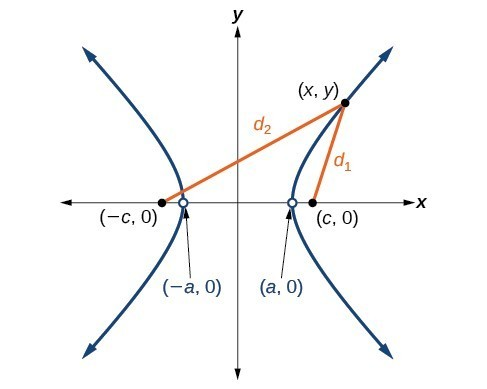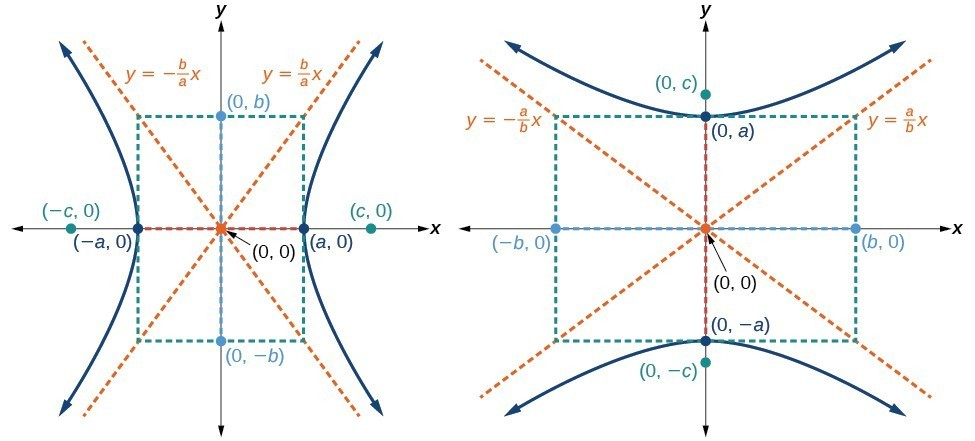## Deriving the Equation of a Hyperbola Centered at the Origin

Let $\left(-c,0\right)$ and $\left(c,0\right)$ be the foci of a hyperbola centered at the origin. The hyperbola is the set of all points $\left(x,y\right)$ such that the difference of the distances from $\left(x,y\right)$ to the foci is constant.Figure 4

If $\left(a,0\right)$ is a vertex of the hyperbola, the distance from $\left(-c,0\right)$ to $\left(a,0\right)$ is $a-\left(-c\right)=a+c$. The distance from $\left(c,0\right)$ to $\left(a,0\right)$ is $c-a$. The sum of the distances from the foci to the vertex is

$\left(a+c\right)-\left(c-a\right)=2a$

If $\left(x,y\right)$ is a point on the hyperbola, we can define the following variables:

$\begin{array}{l}{d}_{2}=\text{the distance from }\left(-c,0\right)\text{ to }\left(x,y\right)\\ {d}_{1}=\text{the distance from }\left(c,0\right)\text{ to }\left(x,y\right)\end{array}$

By definition of a hyperbola, ${d}_{2}-{d}_{1}$ is constant for any point $\left(x,y\right)$ on the hyperbola. We know that the difference of these distances is $2a$ for the vertex $\left(a,0\right)$. It follows that ${d}_{2}-{d}_{1}=2a$ for any point on the hyperbola. As with the derivation of the equation of an ellipse, we will begin by applying the distance formula. The rest of the derivation is algebraic. Compare this derivation with the one from the previous section for ellipses.

$\begin{array}{ll}\text{ }{d}_{2}-{d}_{1}=\sqrt{{\left(x-\left(-c\right)\right)}^{2}+{\left(y - 0\right)}^{2}}-\sqrt{{\left(x-c\right)}^{2}+{\left(y - 0\right)}^{2}}=2a\hfill & \text{Distance Formula}\hfill \\ \sqrt{{\left(x+c\right)}^{2}+{y}^{2}}-\sqrt{{\left(x-c\right)}^{2}+{y}^{2}}=2a\hfill & \text{Simplify expressions}\text{.}\hfill \\ \text{ }\sqrt{{\left(x+c\right)}^{2}+{y}^{2}}=2a+\sqrt{{\left(x-c\right)}^{2}+{y}^{2}}\hfill & \text{Move radical to opposite side}\text{.}\hfill \\ \text{ }{\left(x+c\right)}^{2}+{y}^{2}={\left(2a+\sqrt{{\left(x-c\right)}^{2}+{y}^{2}}\right)}^{2}\hfill & \text{Square both sides}\text{.}\hfill \\ \text{ }{x}^{2}+2cx+{c}^{2}+{y}^{2}=4{a}^{2}+4a\sqrt{{\left(x-c\right)}^{2}+{y}^{2}}+{\left(x-c\right)}^{2}+{y}^{2}\hfill & \text{Expand the squares}\text{.}\hfill \\ \text{ }{x}^{2}+2cx+{c}^{2}+{y}^{2}=4{a}^{2}+4a\sqrt{{\left(x-c\right)}^{2}+{y}^{2}}+{x}^{2}-2cx+{c}^{2}+{y}^{2}\hfill & \text{Expand remaining square}\text{.}\hfill \\ \text{ }2cx=4{a}^{2}+4a\sqrt{{\left(x-c\right)}^{2}+{y}^{2}}-2cx\hfill & \text{Combine like terms}\text{.}\hfill \\ \text{ }4cx - 4{a}^{2}=4a\sqrt{{\left(x-c\right)}^{2}+{y}^{2}}\hfill & \text{Isolate the radical}\text{.}\hfill \\ \text{ }cx-{a}^{2}=a\sqrt{{\left(x-c\right)}^{2}+{y}^{2}}\hfill & \text{Divide by 4}\text{.}\hfill \\ \text{ }{\left(cx-{a}^{2}\right)}^{2}={a}^{2}{\left[\sqrt{{\left(x-c\right)}^{2}+{y}^{2}}\right]}^{2}\hfill & \text{Square both sides}\text{.}\hfill \\ \text{ }{c}^{2}{x}^{2}-2{a}^{2}cx+{a}^{4}={a}^{2}\left({x}^{2}-2cx+{c}^{2}+{y}^{2}\right)\hfill & \text{Expand the squares}\text{.}\hfill \\ \text{ }{c}^{2}{x}^{2}-2{a}^{2}cx+{a}^{4}={a}^{2}{x}^{2}-2{a}^{2}cx+{a}^{2}{c}^{2}+{a}^{2}{y}^{2}\hfill & \text{Distribute }{a}^{2}\text{.}\hfill \\ \text{ }{a}^{4}+{c}^{2}{x}^{2}={a}^{2}{x}^{2}+{a}^{2}{c}^{2}+{a}^{2}{y}^{2}\hfill & \text{Combine like terms}\text{.}\hfill \\ \text{ }{c}^{2}{x}^{2}-{a}^{2}{x}^{2}-{a}^{2}{y}^{2}={a}^{2}{c}^{2}-{a}^{4}\hfill & \text{Rearrange terms}\text{.}\hfill \\ \text{ }{x}^{2}\left({c}^{2}-{a}^{2}\right)-{a}^{2}{y}^{2}={a}^{2}\left({c}^{2}-{a}^{2}\right)\hfill & \text{Factor common terms}\text{.}\hfill \\ \text{ }{x}^{2}{b}^{2}-{a}^{2}{y}^{2}={a}^{2}{b}^{2}\hfill & \text{Set }{b}^{2}={c}^{2}-{a}^{2}.\hfill \\ \text{ }\frac{{x}^{2}{b}^{2}}{{a}^{2}{b}^{2}}-\frac{{a}^{2}{y}^{2}}{{a}^{2}{b}^{2}}=\frac{{a}^{2}{b}^{2}}{{a}^{2}{b}^{2}}\hfill & \text{Divide both sides by }{a}^{2}{b}^{2}\hfill \\ \text{ }\frac{{x}^{2}}{{a}^{2}}-\frac{{y}^{2}}{{b}^{2}}=1\hfill & \hfill \end{array}$

This equation defines a hyperbola centered at the origin with vertices $\left(\pm a,0\right)$ and co-vertices $\left(0\pm b\right)$.

### A General Note: Standard Forms of the Equation of a Hyperbola with Center (0,0)

The standard form of the equation of a hyperbola with center $\left(0,0\right)$ and transverse axis on the x-axis is

$\frac{{x}^{2}}{{a}^{2}}-\frac{{y}^{2}}{{b}^{2}}=1$

where

• the length of the transverse axis is $2a$
• the coordinates of the vertices are $\left(\pm a,0\right)$
• the length of the conjugate axis is $2b$
• the coordinates of the co-vertices are $\left(0,\pm b\right)$
• the distance between the foci is $2c$, where ${c}^{2}={a}^{2}+{b}^{2}$
• the coordinates of the foci are $\left(\pm c,0\right)$
• the equations of the asymptotes are $y=\pm \frac{b}{a}x$

The standard form of the equation of a hyperbola with center $\left(0,0\right)$ and transverse axis on the y-axis is

$\frac{{y}^{2}}{{a}^{2}}-\frac{{x}^{2}}{{b}^{2}}=1$

where

• the length of the transverse axis is $2a$
• the coordinates of the vertices are $\left(0,\pm a\right)$
• the length of the conjugate axis is $2b$
• the coordinates of the co-vertices are $\left(\pm b,0\right)$
• the distance between the foci is $2c$, where ${c}^{2}={a}^{2}+{b}^{2}$
• the coordinates of the foci are $\left(0,\pm c\right)$
• the equations of the asymptotes are $y=\pm \frac{a}{b}x$

Note that the vertices, co-vertices, and foci are related by the equation ${c}^{2}={a}^{2}+{b}^{2}$. When we are given the equation of a hyperbola, we can use this relationship to identify its vertices and foci.Figure 5. (a) Horizontal hyperbola with center $\left(0,0\right)$ (b) Vertical hyperbola with center $\left(0,0\right)$

### How To: Given the equation of a hyperbola in standard form, locate its vertices and foci.

1. Determine whether the transverse axis lies on the x– or y-axis. Notice that ${a}^{2}$ is always under the variable with the positive coefficient. So, if you set the other variable equal to zero, you can easily find the intercepts. In the case where the hyperbola is centered at the origin, the intercepts coincide with the vertices.

a. If the equation has the form $\frac{{x}^{2}}{{a}^{2}}-\frac{{y}^{2}}{{b}^{2}}=1$, then the transverse axis lies on the x-axis. The vertices are located at $\left(\pm a,0\right)$, and the foci are located at $\left(\pm c,0\right)$.

b. If the equation has the form $\frac{{y}^{2}}{{a}^{2}}-\frac{{x}^{2}}{{b}^{2}}=1$, then the transverse axis lies on the y-axis. The vertices are located at $\left(0,\pm a\right)$, and the foci are located at $\left(0,\pm c\right)$.

1. Solve for $a$ using the equation $a=\sqrt{{a}^{2}}$.
2. Solve for $c$ using the equation $c=\sqrt{{a}^{2}+{b}^{2}}$.

### Example 1: Locating a Hyperbola’s Vertices and Foci

Identify the vertices and foci of the hyperbola with equation $\frac{{y}^{2}}{49}-\frac{{x}^{2}}{32}=1$.

### Solution

The equation has the form $\frac{{y}^{2}}{{a}^{2}}-\frac{{x}^{2}}{{b}^{2}}=1$, so the transverse axis lies on the y-axis. The hyperbola is centered at the origin, so the vertices serve as the y-intercepts of the graph. To find the vertices, set $x=0$, and solve for $y$.

$\begin{array}{l}1=\frac{{y}^{2}}{49}-\frac{{x}^{2}}{32}\hfill \\ 1=\frac{{y}^{2}}{49}-\frac{{0}^{2}}{32}\hfill \\ 1=\frac{{y}^{2}}{49}\hfill \\ {y}^{2}=49\hfill \\ y=\pm \sqrt{49}=\pm 7\hfill \end{array}$

The foci are located at $\left(0,\pm c\right)$. Solving for $c$,

$c=\sqrt{{a}^{2}+{b}^{2}}=\sqrt{49+32}=\sqrt{81}=9$

Therefore, the vertices are located at $\left(0,\pm 7\right)$, and the foci are located at $\left(0,9\right)$.

### Try It 1

Identify the vertices and foci of the hyperbola with equation $\frac{{x}^{2}}{9}-\frac{{y}^{2}}{25}=1$.

Solution